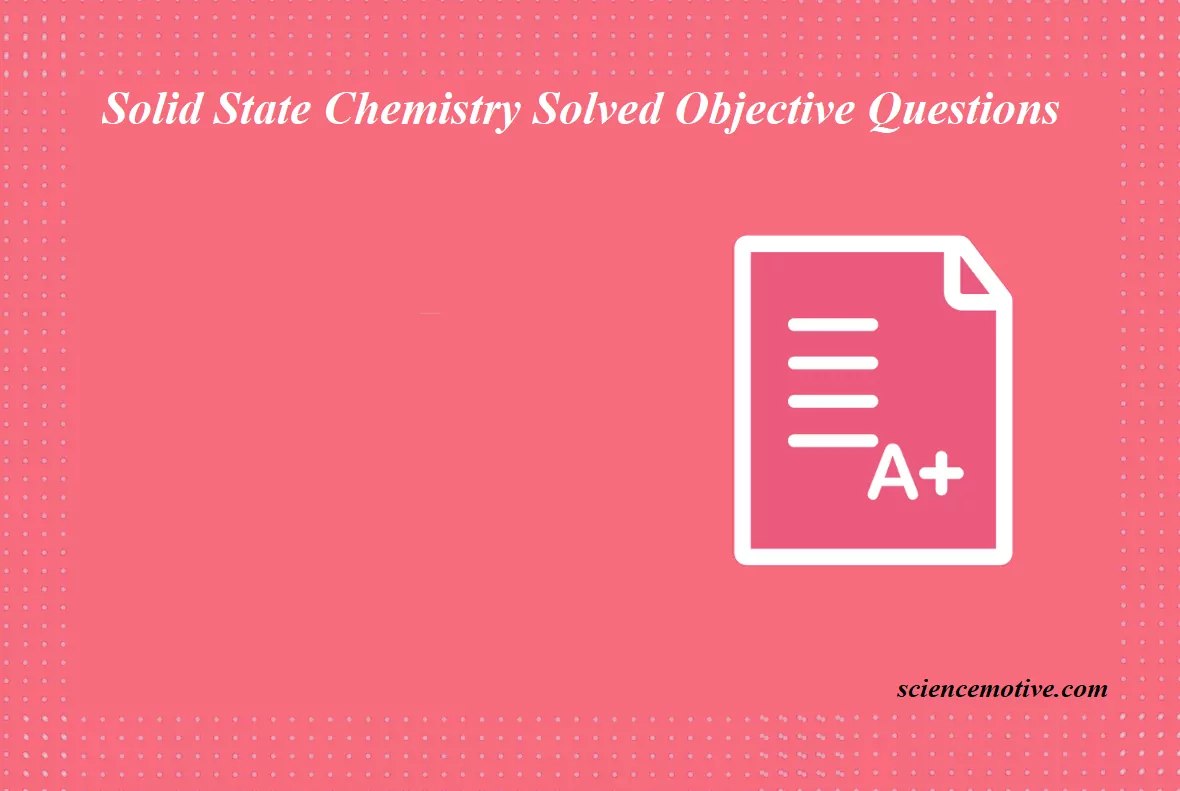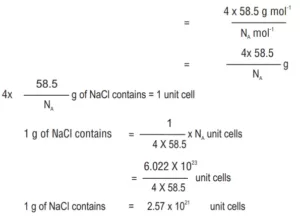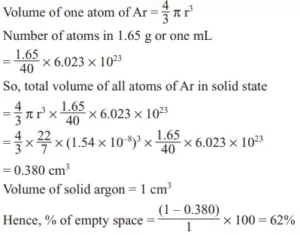# Solid State Chemistry Solved Objective QuestionsSolid State Chemistry Solved Objective Questions

Que 1. Experimentally it was found that a metal oxide has the formula M0.98O1. M is present as M2+ and M3+ in its oxide. Fraction of metal which exists as M3+ would be
a. 7.01%                  b. 4.08%                c. 6.05%                  d. 5.08%
Ans 1. (b)
Explanation: Ratio of the numbers of M atoms to the number of O atoms is
M : O = 0.98 : 1 = 98 : 100
Total +ve charge = Total -ve charge
Because, oxide is neutral
Let, number of M2+ ions = x
So, number of M3+ ions = 98 – x
On equating the charge, we get
2x + 3(98 – x) = -2 (100) (Charge on O atoms) = -200
-x + 294 = 200
x = 94
Number of M2+ ions = 94
Number of M3+ ions = 4
Hence, percentage of M that exists as M3+ = (4/98) × 100 = 4.08 %

Que 2. How many unit cells are present in a cube-shaped ideal crystal of NaCl of mass 1 g?
a. 5.14 × 1021
b. 1.28 × 1021
c. 1.771 × 1021
d. 2.57 × 1021
Ans 2. (d)
Explanation: Mass of the unit cell = Number of formula units in unit cell x mass of each formula unit = 4 × 58.5 g mol-1Solid State Chemistry Solved Objective Questions

Que 3. Which of the following crystals have 6:6 coordination?
(a) MnO                    (b) NH4I                    (c) ZnS                 (d) None of these
Ans 3. (b)
Explanation: NH4I = 6:6                      MnO = 4:4                  ZnS = 4:4

Que 4. A compound contains two types of atoms: X and Y. It crystallizes in a cubic lattice with atoms X at the corners of the unit cell and atoms Y at the body centres. The simplest possible formula of this compound is:
(a) XY                         (b) X2Y2                              (c) XY6                               (d) X8Y
Ans 4. (a)
Explanation: X:Y (8 × 1/8):1(1/1) = 1:1 Therefore, the formula is XY.

Que 5. The number of atoms per unit cell in a BCC, an FCC, and a simple cubic cell is respectively:
(a) 1, 4, 2                    (b) 2, 4, 1                    (c) 4, 1, 2                    (d) 4, 2, 2
Ans 5. (b)

Solid State Chemistry Solved Objective Questions

Que 6. The edge length of the unit cell of NaCl crystal lattice is 552 pm. If the ionic radius of sodium ion is 95 pm. What is the ionic radius of chloride ions?
(a) 181 pm                  (b) 190 pm                  (c) 276 pm                  (d) 368 pm
Ans 6. (a)
Explanation: The edge length of unit cell of NaCl = 552 pm
The ionic radius of sodium ion = 95 pm
The ionic radius of Cl ion = (552/2) – 95
276 – 95 = 181 pm

Que 7. The density of solid argon is 1.65 g/mL at –233°C. If the argon atom is assumed to be a sphere of radius 1.54 × 10–8 cm, what percentage of solid argon is apparently empty space? (Atomic wt. of Ar = 40)
(a) 32%                         (b) 52%                       (c) 62%                       (d) 72%
Ans 7. (c)
Explanation:Solid State Chemistry Solved Objective Questions

Que 8. A solid has 3 types of atoms namely X, Y, and Z. X forms an FCC lattice with Y atoms occupying all the tetrahedral voids and Z atoms occupying half the octahedral voids. The formula of the solid is
(a) XYZ                        (b) X2Y4Z                      (c) X4YZ2                      (d) X4Y2Z
Ans 8. (b)
Explanation: X (FCC) = 8 × 1/8 = 1, that is, 1
Y (tetrahedral voids) = 2,
that is, 2 Z (octahedral voids) = 1, that is, ½
X : Y : Z = 1 : 2 : 1/2 = 2 : 4 : 1
= X2Y4Z

Que 9. Amorphous substances show:
(1) Short and long-range order
(2) Short-range order
(3) Long-range order
(4) Have no sharp melting point
(a) (1) and (2) are correct                              (b) (2) and (4) are correct
(c) (2), (3) and (4) are correct                        (d) (1) and (4) are correct
Ans 9. (b)

Que 10. Na2O has an antifluorite structure. In Na2O, the coordination number of Na+ and O2– are respectively:
(a) 4, 4                   (b) 6, 6                     (c) 4, 8                                (d) 8, 8
Ans 10. (c)
Explanation: Antifluorite structures like Li2O, Na2O, K2O, Rb2O, Rb2S, Na2S possess 4:8 coordination.

Solid State Chemistry Solved Objective Questions